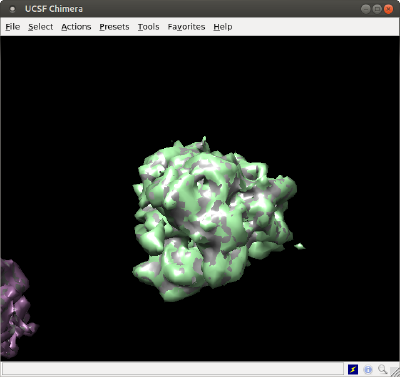# Walkthrough on placement of averages on table positions

In this walkthrough we show:

• how to create a triangulation that represents a density map,
• how to repeat it at the positions and orientations expressed by a Dynamo table (placement operation)
• how to visualize the same scene in Chimera:
1. through a single model for each copy of the density map
2. through a single mesh for the full scene

## Creation of a density map

We will just use the ribosome32.em template inside your Dynamo installation.

```%
% We create a tomogram with multiple copies of a ribosome template
%

% just a normalization operation to
rbright = dynamo_normalize_roi(r);

% inverts and normalizesit
% protein is thus dark on bright ground

% prepare a black (zeros) volume of the size of the template

% we select a threshold
threshold = - 0.1;

% and ellimiate densities below it
includedIndices = find(rbright>threshold);

% we show the density map as a series of orthoslices
dview(rbright);

```

Now, our task is to create a triangulation (a mesh) that represents an isosurface to be rendered later. You could just use Chimera for this, by opening the map, choosing your threshold level visually and exporting and .stl file. Alternatively, you can do it directly form the Dynamo command line with the dynamo_isosurface command.

## Polishing the template triangulation

```Here we show some functions in the <tt>mbgeom.triangulation</tt> package that can be used to polish the mesh.

%%
%
% Now, we create an STL file out of the template
%

% this is a dTriRep object that covers a triangulation
% its simply a convenient wrapper around a matlab 'triangulation' object)
isolevel = 6.2;

% we can even create a smoother surface
tSmooth = mbgeom.triangulation.smoothingSubdivision(dt.tr,'mr',1);

% the smoothing algorithm sometimes creates peaks, which can just be
% removed
tClean =  mbgeom.triangulation.removePeaks(tSmooth,'std',2);

% unconnected pieces can also be removed
tNoDust=  mbgeom.triangulation.removeDust(tClean);

% [optional]
% if we want to export a triangulation to 3D design softwares, we should
% impose a consistent orientation for the normals of the triangles
tFinal = mbgeom.triangulation.consistentNormals(tNoDust,'alignTo','out');

% Let's see what we just created:
figure; f = gcf; f.Name = 'construction of ribosome template';

triangulationsToPlot = {dt.tr,tSmooth,tClean,tNoDust};
titles               = {'direct triangulation','smoothed','peaks removed','dust removed'};
for i=1:4;
ax(i) =subplot(2,2,i);
h(i) = trisurf(triangulationsToPlot{i});
axis('equal');
h(i).LineStyle = 'none';
lightangle(-45,30);
axis(ax(i),'off');
ht(i) = title(ax(i),titles{i});
ht(i).Color = 'w';
mbgraph.cursors.setMouse3d(ax(i));
end

f.Color = 'k';

```

## Repeating the density map along table positions

### Create table

We create first a table with random positions:

```%
% table random locations for the template
%

Nribosomes = 20;
lx = 300;
ly = 300;
lz = 400;
tb = dynamo_table_random(Nribosomes,'ts',60,'lx',300,'ly',300,'lz',300);

% we elliminate table positions that are too close to each other
tb=dpktbl.exclusionPerVolume(tb,60);

% uses round coordinates for placement of maps
tb(:,24:26) = round(tb(:,24:26));

```

### Place mesh on table

We use to place the triangulation on the different locations of the table.

Important: you need to pass the effective rotation center of the triangulation, i.e, the point about which the rotations in the table are defined. For a volume of 32 x 32 x 32, the center is 16.5*[1,1,1];

```tAll = dpktbl.triangulation.place(tb,tFinal,'rc',16.5);

```

### Depict table

Now, tAll contains a single mesh with all the ribosomes. You can depict it inside Dynamo, which allows you use further Dynamo matlab tools to enrich the scene on a later point.

```%%
f=figure; haxis = gca();
%ormaps and graymaps in the same plot
dynamo_colormap();
h = trisurf(tAll);
h.LineStyle = 'none';
axis equal;
f.Name = 'Simple trisurf scene';

% just make the explroation
mbgraph.cursors.setMouse3d([]);

% We can the play with the representation

l = lightangle(-45,30);

```

Note that you can make the illumination rotate with the viewing perspective if you rotate it by moving the cursor while keeping the main button pressed. If you use instead Matlab's rotation icon, the defined light will remain fixed while you rotate.

## Depiction in Chimera

### As single surface file

The created triangulation can be directly exported:

```dynamo_write_stl(tAll,'singleMesh.stl');

```

and then you can open it directly in Chimera. In a operative system shell (not a Matlab/Dynamo shell), just write:

``` chimera singleMesh.stl &

```

### As several volume models

The basic command is dtchimera. With the code below, we create a .cmd file that will open one model for one ribosome.

```dtchimera(tb,'-template',rbright,'-show',1,'level',isolevel,'open','singleMesh.stl');

```

In order to check how this representation overlays with the previous one, we are using the same starting isolevel for the individual models, and also we are passing the order to Chimera to open the surface that we created with the previous method, just for comparative purposes.

Note that both depictions are equivalent, as we have used the same isolevel for both of them.Zoomed view on one model: green is the model produced by chimera, silver is the isosurface created by Dynamo.